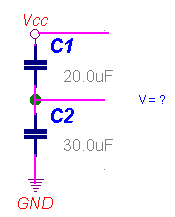HITEQUEST technical interview Q & A for high-tech professionals

Interview Questions
Electronics Hardware
Computer Software
Quick Thinking Tests
General questions
Phone screening questions
Submit your Q or A

Resources
Technical articles
Technical discussion
Resume and interview
How to get a job in Silicon Valley
How much are you worth on market?
Why you may need an agent

Break point
Written Exam
Logic Tests
Professional Test
Tomato company
Cup of coffee
How stock market works
Engineering jokes

About Hitequest
About Hitequest
Home page

# =Electronics Hardware Questions=Q:Sent by Tanh, VLSI engineer
What will be the voltage level between the two capacitors? The Vcc = 10v DC.Hint from Hitequest:
Q1= V1*C1      //ammount of charge on C1
Q2= V2*C2      //ammount of charge on C2
Q = Q1 = Q2      //all caps in series have the same charge
(V-V2)*C1=V2*C2
V2=V*C1/(C1+C2)
V2=10*20/50=4V     //the result

Hector M.:
there is an error on the question with the 2 capacitors in series (20uf and 30uf)....
The solution is 6 volts instead of 4 volts. The voltage divider formula is:
V=(C2.Vcc)/(C1+C2)

Marvin:
My comment is related to the question sent by Tanh. As shown in the figure the required voltage is the voltage on the capacitor C2 (The voltage is always measured between two points). The response shown is correct.

Hector M comment is wrong - The voltage divider for the capacitors is V2= V*C1/(C1+C2) which obviously is not as shown by him.
His formula is an extension of the resistor voltage divider, V2=V*R2/(R1+R2)where R1 and R2 are the resistors replacing the capacitors in a resistor network.

anonym:
Tanh's problem about two capacitors in series is under-specified. If the capacitors start out discharged, then they will charge up as indicated since the current is the same through the circuit and therefore q1 = q2.
However, if the capacitors have some initial charge, that changes everything.
One can imagine a stable condition in which, for instance, V1 = 9V and V2 = 1V.
The net charge across each capacitor equals its own voltage, so no current flows.